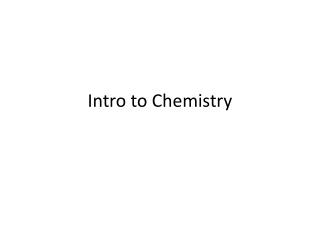DownloadDownload PresentationIntro to Chemistry

# Intro to Chemistry

Download Presentation## Intro to Chemistry

- - - - - - - - - - - - - - - - - - - - - - - - - - - E N D - - - - - - - - - - - - - - - - - - - - - - - - - - -
##### Presentation Transcript

1. Intro to Chemistry

2. Scientific Notation • Review your handout from before • 10 = 1 x 101 • 1000 = 1 X 103 = 10 x 10 x 10 • .1 = 10-1 = 1/10 • .01 = 10-2 = 1/100 = 1/102 • .001 = 10-3 = 1/1000 = 1/103 • 1 = 10 • 2000 = 2(1000) = 2 x 103 ↑ • any number between 1-10 = 10+or-

3. 600,000 = 6 x 105 • 17,000,000 = 1.7 x 107 • 602200000000000000000000 = 6.022 x 1023 • 2070000000 = _______________ • .0036 = ___________________ • .00000512 = _________________ • .00000000085 = ______________

4. Multiplying using exponents = add them together • 106 x102 = 108 • 2 x 104 x 3 x 103 = 6 x 107 • (5 x 102) (7 x 105) = 35 x 107 = 3.5 x 108 ↑ only 1 number before the decimal in scientific notation

5. Dividing Exponents = subtract them • 105 / 103= 102 • 8 x 105 / 2 x 109 = 4 x 10-4 • If you are adding, 102 + 103, do not add unlike exponents • Instead, convert back to original form then add • So, 102 = 100 and 103 = 1000 which = 100 + 1000 = 1100 = 1.1 x 103